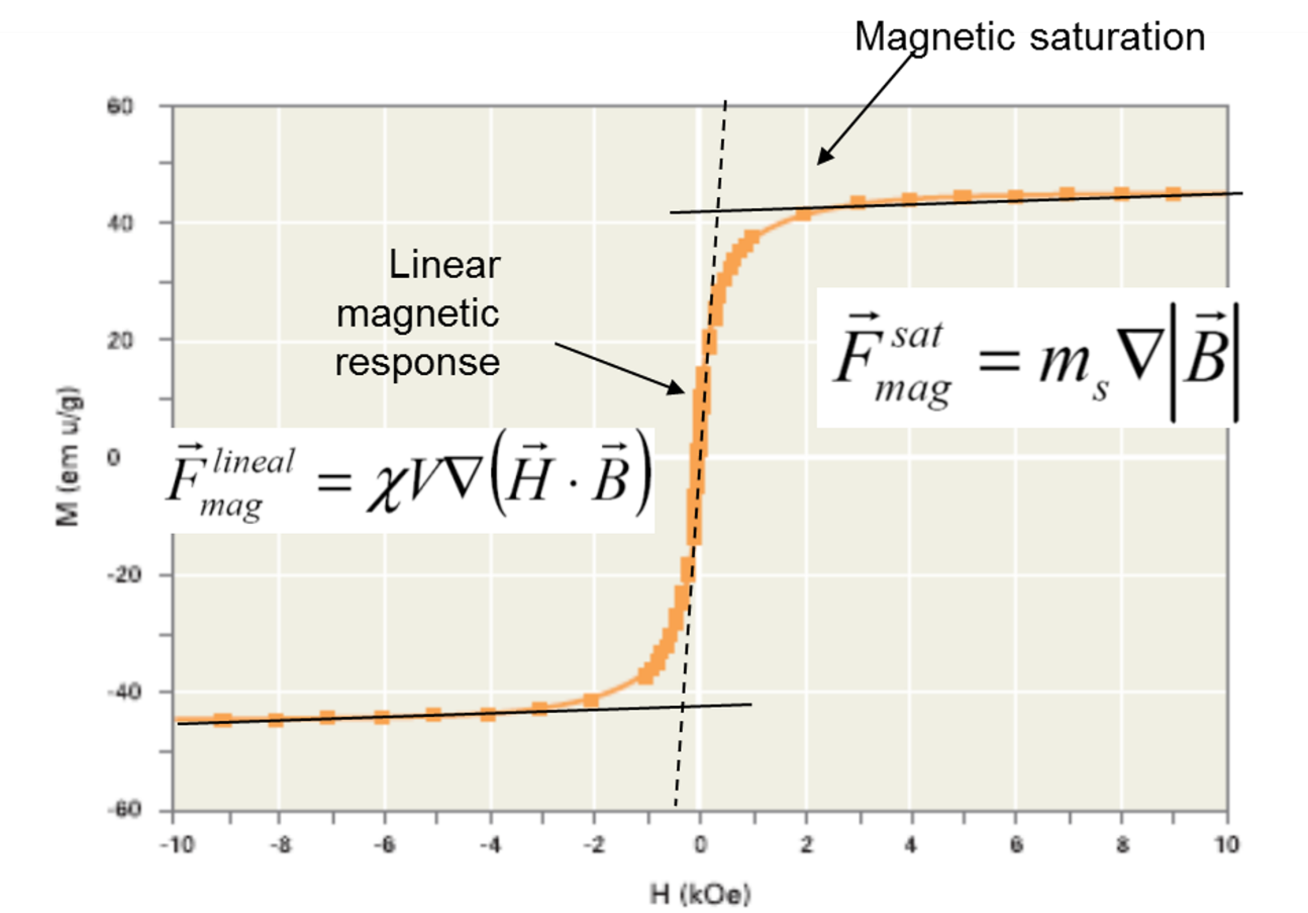Blog

A constant magnetic force across the whole working volume is key to consistency in biomagnetic separation processes. This ensures that all beads in the suspension experience the same force. Classical magnetic separators can't provide these conditions because the magnetic force they generate decreases with distance.

The generic magnetic force expression is the gradient of the scalar product of the magnetic moment of the bead and the magnetic field. For magnetic beads, if their magnetic moment aligns with the applied magnetic field, then both vectors are parallel. This allows magnetic force to be expressed differently when the magnetic field is low or high.

## When the magnetic field is low

Magnetic susceptibility is the ratio between magnetization and the applied magnetic field. When magnetic susceptibility is constant, the magnetic force will be proportional to the gradient of the square of the applied field. This is why, in some of the literature, magnetic force is expressed as T2/m. The authors implicitly assume that the magnetic beads are not saturated.

To get a constant magnetic force in these conditions, you need to have a magnetic field with an intensity that varies with the square root of the distance. This kind of magnetic field profile is complex (if not impossible) to generate.Magnetic force expression at low applied magnetic field (constant susceptibility, linear response) and at high magnetic field (magnetic saturation, constant magnetic moment).

## When the magnetic field is high

When the beads are magnetically saturated, as the magnetic field increases, the magnetic response is no longer linear. For even higher magnetic field values, the magnetic moment of the beads remains close to its saturation value. Here, if we can assume the magnetic moment is constant, then the magnetic force is directly proportional to the magnetic field gradient.

The following two conditions must be met to maintain a constant magnetic force in biomagnetic separation processes:

1. The magnetic field needs to vary linearly with the distance of the beads from the magnet.
2. The beads should be magnetically saturated so that the field is high enough (e.g., B < 0.1 T for magnetite).

Newer, more advanced constant magnetic biomagnetic separation systems such as Sepmag fulfill these two conditions at virtually any volume. This is possible because these systems have a constant radial magnetic field in the core. In these systems, the gradient is adjusted so that the magnetic field is over 0.1 T everywhere except in a small area around the axis. All beads, therefore, experience the same force and move at the same radial speed.

As such, for advanced biomagnetic separation systems, the force is constant and well-defined so scaling up is typically straightforward. To successfully scale up your biomagnetic separation processes, you need to carefully consider the exact conditions of your system (e.g., variation of the magnetic field, characteristics of the beads, and characteristics of the magnetic field). When scaling up your process, you must scale up the magnetic force, not the magnetic field.

The old saying ‘magnetic beads don’t work at large volumes’ is, simply, wrong. If you want to learn what leading IVD-manufacturers already know, this is the e-book for you!Related news